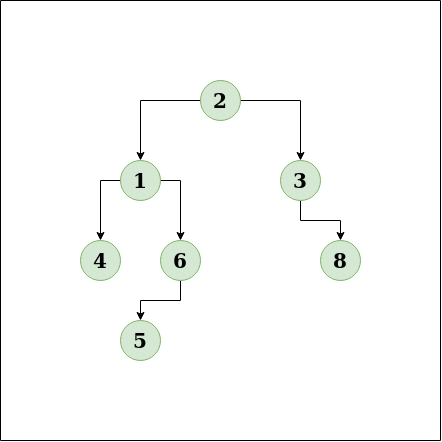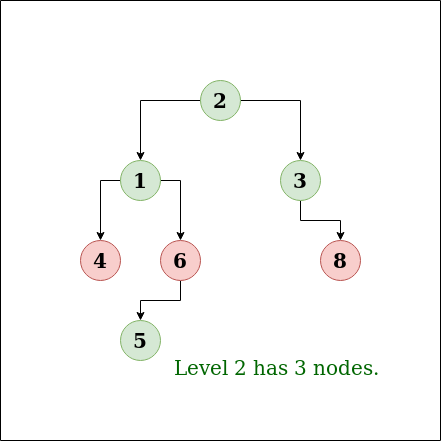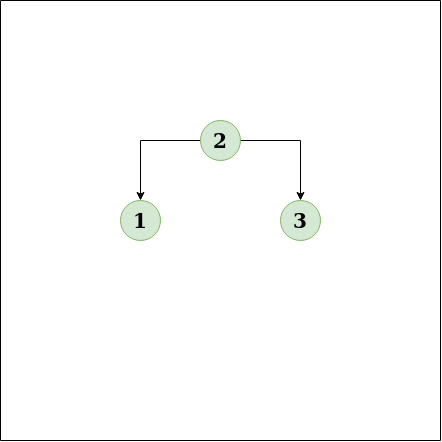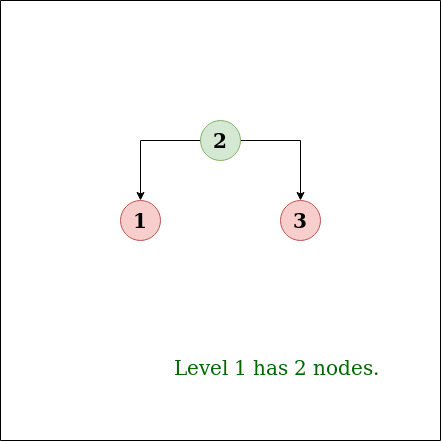# Level with maximum number of nodes

Find the level in a binary tree which has the maximum number of nodes. The root is at level 0.

Examples:

```Input:Output : 2
Explanation:Input:Output:1
Explanation```

## Recommended: Please try your approach on {IDE} first, before moving on to the solution.

Approach: It is known that in level order traversal of binary tree with queue, at any time our queue contains all elements of a particular level. So find level with maximum number of nodes in queue.
BFS traversal is an algorithm for traversing or searching tree or graphs . It starts at the tree root , and explores all of the neighbor nodes at the present depth prior to moving on to the nodes at the next depth level.
So at any point the queue of BFS will contain elements of adjacent layers. So this makes the algorithm perfect for this problem.

Algorithm:

1. Create the tree, a queue to store the nodes and insert the root in the queue. Create variables level=0,count =0 and level_no=-1
2. The implementation will be slightly different, all the elements of same level will be removed in a single iteration.
3. Run a loop while size of queue is greater than 0. Get the size of queue (size) and store it. If size is greater than count then update count = size and level_no = level.
4. Now run a loop size times, and pop one node from the queue and insert its childrens (if present).
5. Increment level.

Implementation:

## C++

 `// C++ implementation to find the level ` `// having maximum number of Nodes ` `#include ` `using` `namespace` `std; ` ` `  `/* A binary tree Node has data, pointer ` `   ``to left child and a pointer to right ` `   ``child */` `struct` `Node ` `{ ` `    ``int` `data; ` `    ``struct` `Node* left; ` `    ``struct` `Node* right; ` `}; ` ` `  `/* Helper function that allocates a new node with the ` `   ``given data and NULL left and right pointers. */` `struct` `Node* newNode(``int` `data) ` `{ ` `    ``struct` `Node* node = ``new` `Node; ` `    ``node->data = data; ` `    ``node->left = NULL; ` `    ``node->right = NULL; ` `    ``return``(node); ` `} ` ` `  `// function to find the level ` `// having maximum number of Nodes ` `int` `maxNodeLevel(Node *root) ` `{ ` `    ``if` `(root == NULL) ` `        ``return` `-1; ` ` `  `    ``queue q; ` `    ``q.push(root); ` ` `  `    ``// Current level ` `    ``int` `level = 0; ` ` `  `    ``// Maximum Nodes at same level ` `    ``int` `max = INT_MIN; ` ` `  `    ``// Level having maximum Nodes ` `    ``int` `level_no = 0; ` ` `  `    ``while` `(1) ` `    ``{ ` `        ``// Count Nodes in a level ` `        ``int` `NodeCount = q.size(); ` ` `  `        ``if` `(NodeCount == 0) ` `            ``break``; ` ` `  `        ``// If it is maximum till now ` `        ``// Update level_no to current level ` `        ``if` `(NodeCount > max) ` `        ``{ ` `            ``max = NodeCount; ` `            ``level_no = level; ` `        ``} ` ` `  `        ``// Pop complete current level ` `        ``while` `(NodeCount > 0) ` `        ``{ ` `            ``Node *Node = q.front(); ` `            ``q.pop(); ` `            ``if` `(Node->left != NULL) ` `                ``q.push(Node->left); ` `            ``if` `(Node->right != NULL) ` `                ``q.push(Node->right); ` `            ``NodeCount--; ` `        ``} ` ` `  `        ``// Increment for next level ` `        ``level++; ` `    ``} ` ` `  `    ``return` `level_no; ` `} ` ` `  `// Driver program to test above ` `int` `main() ` `{ ` `    ``// binary tree formation ` `    ``struct` `Node *root = newNode(2);      ``/*        2      */` `    ``root->left        = newNode(1);      ``/*      /   \    */` `    ``root->right       = newNode(3);      ``/*     1     3      */` `    ``root->left->left  = newNode(4);      ``/*   /   \    \  */` `    ``root->left->right = newNode(6);      ``/*  4     6    8 */` `    ``root->right->right  = newNode(8);    ``/*       /       */` `    ``root->left->right->left = newNode(5);``/*      5        */` ` `  `    ``printf``(``"Level having maximum number of Nodes : %d"``, ` `            ``maxNodeLevel(root)); ` `    ``return` `0; ` `} `

## Java

 `// Java implementation to find the level  ` `// having maximum number of Nodes  ` `import` `java.util.*; ` `class` `GfG {  ` ` `  `/* A binary tree Node has data, pointer  ` `to left child and a pointer to right  ` `child */` `static` `class` `Node  ` `{  ` `    ``int` `data;  ` `    ``Node left;  ` `    ``Node right;  ` `} ` ` `  `/* Helper function that allocates a new node with the  ` `given data and NULL left and right pointers. */` `static` `Node newNode(``int` `data)  ` `{  ` `    ``Node node = ``new` `Node();  ` `    ``node.data = data;  ` `    ``node.left = ``null``;  ` `    ``node.right = ``null``;  ` `    ``return``(node);  ` `}  ` ` `  `// function to find the level  ` `// having maximum number of Nodes  ` `static` `int` `maxNodeLevel(Node root)  ` `{  ` `    ``if` `(root == ``null``)  ` `        ``return` `-``1``;  ` ` `  `    ``Queue q = ``new` `LinkedList ();  ` `    ``q.add(root);  ` ` `  `    ``// Current level  ` `    ``int` `level = ``0``;  ` ` `  `    ``// Maximum Nodes at same level  ` `    ``int` `max = Integer.MIN_VALUE;  ` ` `  `    ``// Level having maximum Nodes  ` `    ``int` `level_no = ``0``;  ` ` `  `    ``while` `(``true``)  ` `    ``{  ` `        ``// Count Nodes in a level  ` `        ``int` `NodeCount = q.size();  ` ` `  `        ``if` `(NodeCount == ``0``)  ` `            ``break``;  ` ` `  `        ``// If it is maximum till now  ` `        ``// Update level_no to current level  ` `        ``if` `(NodeCount > max)  ` `        ``{  ` `            ``max = NodeCount;  ` `            ``level_no = level;  ` `        ``}  ` ` `  `        ``// Pop complete current level  ` `        ``while` `(NodeCount > ``0``)  ` `        ``{  ` `            ``Node Node = q.peek();  ` `            ``q.remove();  ` `            ``if` `(Node.left != ``null``)  ` `                ``q.add(Node.left);  ` `            ``if` `(Node.right != ``null``)  ` `                ``q.add(Node.right);  ` `            ``NodeCount--;  ` `        ``}  ` ` `  `        ``// Increment for next level  ` `        ``level++;  ` `    ``}  ` ` `  `    ``return` `level_no;  ` `}  ` ` `  `// Driver program to test above  ` `public` `static` `void` `main(String[] args)  ` `{  ` `    ``// binary tree formation  ` `     ``Node root = newNode(``2``);     ``/*     2     */` `    ``root.left     = newNode(``1``);     ``/*     / \ */` `    ``root.right     = newNode(``3``);     ``/*     1     3     */` `    ``root.left.left = newNode(``4``);     ``/* / \ \ */` `    ``root.left.right = newNode(``6``);     ``/* 4     6 8 */` `    ``root.right.right = newNode(``8``); ``/*     /     */` `    ``root.left.right.left = newNode(``5``);``/*     5     */` ` `  `    ``System.out.println(``"Level having maximum number of Nodes : "` `+ maxNodeLevel(root));  ` `} ` `}  `

## Python3

 `# Python3 implementation to find the  ` `# level having Maximum number of Nodes ` ` `  `# Importing Queue ` `from` `queue ``import` `Queue  ` ` `  `# Helper class that allocates a new  ` `# node with the given data and None ` `# left and right pointers.  ` `class` `newNode: ` `    ``def` `__init__(``self``, data): ` `        ``self``.data ``=` `data  ` `        ``self``.left ``=` `None` `        ``self``.right ``=` `None` ` `  `# function to find the level  ` `# having Maximum number of Nodes  ` `def` `maxNodeLevel(root): ` `    ``if` `(root ``=``=` `None``):  ` `        ``return` `-``1` ` `  `    ``q ``=` `Queue()  ` `    ``q.put(root)  ` ` `  `    ``# Current level  ` `    ``level ``=` `0` ` `  `    ``# Maximum Nodes at same level  ` `    ``Max` `=` `-``999999999999` ` `  `    ``# Level having Maximum Nodes  ` `    ``level_no ``=` `0` ` `  `    ``while` `(``1``): ` `         `  `        ``# Count Nodes in a level  ` `        ``NodeCount ``=` `q.qsize()  ` ` `  `        ``if` `(NodeCount ``=``=` `0``): ` `            ``break` ` `  `        ``# If it is Maximum till now  ` `        ``# Update level_no to current level  ` `        ``if` `(NodeCount > ``Max``): ` `            ``Max` `=` `NodeCount  ` `            ``level_no ``=` `level ` ` `  `        ``# Pop complete current level  ` `        ``while` `(NodeCount > ``0``): ` `            ``Node ``=` `q.queue[``0``]  ` `            ``q.get() ` `            ``if` `(Node.left !``=` `None``): ` `                ``q.put(Node.left)  ` `            ``if` `(Node.right !``=` `None``):  ` `                ``q.put(Node.right)  ` `            ``NodeCount ``-``=` `1` ` `  `        ``# Increment for next level  ` `        ``level ``+``=` `1` ` `  `    ``return` `level_no ` ` `  `# Driver Code ` `if` `__name__ ``=``=` `'__main__'``: ` `     `  `    ``# binary tree formation  ` `    ``root ``=` `newNode(``2``)     ``#     2      ` `    ``root.left     ``=` `newNode(``1``)     ``#     / \  ` `    ``root.right     ``=` `newNode(``3``)     ``#     1     3      ` `    ``root.left.left ``=` `newNode(``4``)     ``# / \ \  ` `    ``root.left.right ``=` `newNode(``6``)     ``# 4     6 8  ` `    ``root.right.right ``=` `newNode(``8``) ``#     /      ` `    ``root.left.right.left ``=` `newNode(``5``)``#     5      ` ` `  `    ``print``(``"Level having Maximum number of Nodes : "``,  ` `                                 ``maxNodeLevel(root)) ` ` `  `# This code is contributed by Pranchalk `

## C#

 `using` `System; ` `using` `System.Collections.Generic; ` ` `  `// C# implementation to find the level   ` `// having maximum number of Nodes   ` `public` `class` `GfG ` `{ ` ` `  `/* A binary tree Node has data, pointer   ` `to left child and a pointer to right   ` `child */` `public` `class` `Node ` `{ ` `    ``public` `int` `data; ` `    ``public` `Node left; ` `    ``public` `Node right; ` `} ` ` `  `/* Helper function that allocates a new node with the   ` `given data and NULL left and right pointers. */` `public` `static` `Node newNode(``int` `data) ` `{ ` `    ``Node node = ``new` `Node(); ` `    ``node.data = data; ` `    ``node.left = ``null``; ` `    ``node.right = ``null``; ` `    ``return` `(node); ` `} ` ` `  `// function to find the level   ` `// having maximum number of Nodes   ` `public` `static` `int` `maxNodeLevel(Node root) ` `{ ` `    ``if` `(root == ``null``) ` `    ``{ ` `        ``return` `-1; ` `    ``} ` ` `  `    ``LinkedList q = ``new` `LinkedList (); ` `    ``q.AddLast(root); ` ` `  `    ``// Current level   ` `    ``int` `level = 0; ` ` `  `    ``// Maximum Nodes at same level   ` `    ``int` `max = ``int``.MinValue; ` ` `  `    ``// Level having maximum Nodes   ` `    ``int` `level_no = 0; ` ` `  `    ``while` `(``true``) ` `    ``{ ` `        ``// Count Nodes in a level   ` `        ``int` `NodeCount = q.Count; ` ` `  `        ``if` `(NodeCount == 0) ` `        ``{ ` `            ``break``; ` `        ``} ` ` `  `        ``// If it is maximum till now   ` `        ``// Update level_no to current level   ` `        ``if` `(NodeCount > max) ` `        ``{ ` `            ``max = NodeCount; ` `            ``level_no = level; ` `        ``} ` ` `  `        ``// Pop complete current level   ` `        ``while` `(NodeCount > 0) ` `        ``{ ` `            ``Node Node = q.First.Value; ` `            ``q.RemoveFirst(); ` `            ``if` `(Node.left != ``null``) ` `            ``{ ` `                ``q.AddLast(Node.left); ` `            ``} ` `            ``if` `(Node.right != ``null``) ` `            ``{ ` `                ``q.AddLast(Node.right); ` `            ``} ` `            ``NodeCount--; ` `        ``} ` ` `  `        ``// Increment for next level   ` `        ``level++; ` `    ``} ` ` `  `    ``return` `level_no; ` `} ` ` `  `// Driver program to test above   ` `public` `static` `void` `Main(``string``[] args) ` `{ ` `    ``// binary tree formation   ` `     ``Node root = newNode(2); ``//  2 ` `    ``root.left = newNode(1); ``//  / \ ` `    ``root.right = newNode(3); ``//  1   3 ` `    ``root.left.left = newNode(4); ``// / \ \ ` `    ``root.left.right = newNode(6); ``// 4    6 8 ` `    ``root.right.right = newNode(8); ``//    / ` `    ``root.left.right.left = newNode(5); ``//     5 ` ` `  `    ``Console.WriteLine(``"Level having maximum number of Nodes : "` `+ maxNodeLevel(root)); ` `} ` `} ` ` `  `// This code is contributed by Shrikant13 `

Output:

```Level having maximum number of nodes : 2
```

Complexity Analysis:

• Time Complexity : O(n).
In BFS traversal every node is visited only once, So Time Complexity is O(n).
• Space Complexity: O(n).
The space is required to store the nodes in a queue.

This article is contributed by Ayush Jauhari. If you like GeeksforGeeks and would like to contribute, you can also write an article using contribute.geeksforgeeks.org or mail your article to contribute@geeksforgeeks.org. See your article appearing on the GeeksforGeeks main page and help other Geeks.

Attention reader! Don’t stop learning now. Get hold of all the important DSA concepts with the DSA Self Paced Course at a student-friendly price and become industry ready.

My Personal Notes arrow_drop_up

Article Tags :
Practice Tags :

5

Please write to us at contribute@geeksforgeeks.org to report any issue with the above content.